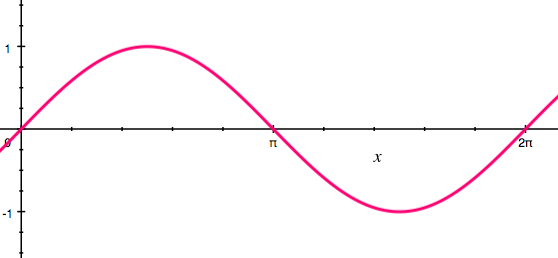# Elementary Taylor Series

Calculus Level 1Compute the first three non-zero terms of the Taylor series for $f(x) = \sin x$ centered at $x = 0.$

×